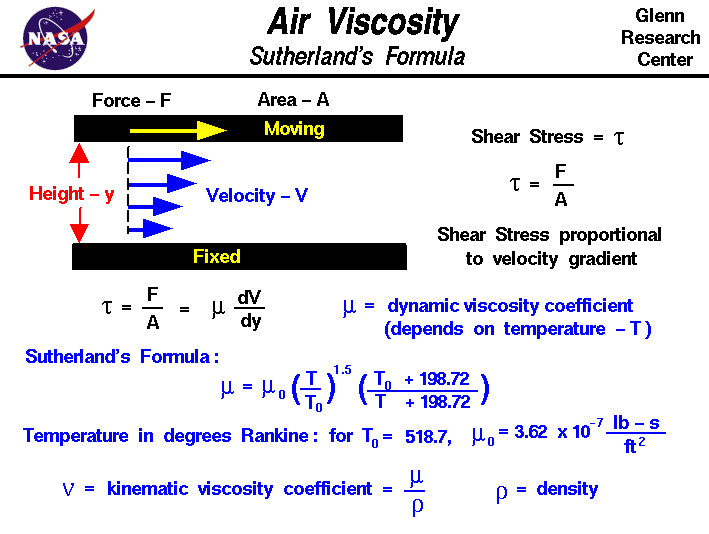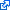+ Text Only Site
+ Non-Flash Version
+ Contact GlennAs an object moves through a gas, the gas molecules near the object are disturbed and move around the object. Aerodynamic forces are generated between the gas and the object. The magnitude of these forces depend on the shape of the object, the speed of the object, the mass of the gas going by the object and on two other important properties of the gas; the viscosity, or stickiness, of the gas and the compressibility, or springiness, of the gas. To properly model these effects, aerodynamicists use similarity parameters which are ratios of these effects to other forces present in the problem. If two experiments have the same values for the similarity parameters, then the relative importance of the forces are being correctly modeled. Representative values for the properties of air are given on another page, but the actual value of the parameter depends on the state of the gas and on the altitude. On this page, we examine the viscosity of a gas.

As an object moves through a gas, the gas molecules stick to the surface. If we have two surfaces, as shown in the figure, with one surface fixed and the other surface moving parallel to the fixed surface, a shearing force is generated in the fluid between the surfaces. An external force F must be applied to the moving surface to overcome the resistance of the fluid. If we denote the magnitude of the shearing force by the Greek letter tau. Then:

tau = F / A

where A is the area of the moving surface. It is experimentally observed that, for most gases, the shear stress is directly proportional to the gradient of the velocity between the surfaces:

tau is linearly proportional to dV/dy

where y is the distance between the surfaces and V is the velocity. The proportionality constant is called the coefficient of dynamic viscosity and assigned the Greek letter mu

tau = (mu) * (dV/dy)

The value of the dynamic viscosity coefficient is found to be a constant with pressure but the value depends on the temperature of the gas. For air, D. M. Sutherland provides an equation for the dependence on temperature T:

mu = mu0 * ((T / T0)^1.5) * ((T0 + 198.72) / (T + 198.72))

where mu0 and T0 are reference values given at sea level stanfard conditions. The temperature is specified in degrees Rankine:

mu0 = 3.62 x 10^-7 lb-sec/ft^2

T0 = 518.7 R

The shearing of the flow of air creates a layer of air near the surface, called a boundary layer, which, in effect, changes the shape of the object. The flow of gas reacts to the edge of the boundary layer as if it was the physical surface of the object. To make things more confusing, the boundary layer may separate from the body and create an effective shape much different from the physical shape. And to make it even more confusing, the flow conditions in and near the boundary layer are often unsteady (changing in time). The boundary layer is very important in determining the drag of an object. To determine and predict these conditions, aerodynamicists rely on wind tunnel testing and very sophisticated computer analysis.

The similarity parameter for viscosity is the Reynolds number. The Reynolds number expresses the ratio of inertial forces to viscous forces. From a detailed analysis of the momentum conservation equation, the inertial forces are characterized by the product of the density r times the velocity V times the gradient of the velocity dV/dx. The viscous forces are characterized by the viscosity coefficient mu times the second gradient of the velocity d^2V/dx^2. The Reynolds number Re then becomes:

Re = (r * V * dV/dx) / (mu * d^2V/dx^2)

Re = (r * V * L) / mu

where L is some characteristic length of the problem. If the Reynolds number of the experiment and flight are close, then we properly model the effects of the viscous forces relative to the inertial forces. If they are very different, we do not correctly model the physics of the real problem and predict incorrect levels of the aerodynamic forces.

The dynamic viscosity coefficient divided by the density is called the kinematic viscosity and given the Greek symbol nu

nu = mu / r

Re = V * L / nu

The units of nu are length^2/sec.

Here's a JavaScript program to calculate the coefficient of viscosity and the Reynolds number for different altitude, length, and speed.

Due to IT security concerns, many users are currently experiencing problems running NASA Glenn educational applets. The applets are slowly being updated, but it is a lengthy process. If you are familiar with Java Runtime Environments (JRE), you may want to try downloading the applet and running it on an Integrated Development Environment (IDE) such as Netbeans or Eclipse. The following are tutorials for running Java applets on either IDE:
NetbeansEclipse# Similarity Parameter Calculator

Please Input Altitude, Speed, and Length Scale

Input
feet
mph
feet
Output
Speed/Mach Number
Speed
Speed of Sound
Dynamic Press
Mach #
Compressibility
P static
P total
T static
T total
Viscosity
Density
Dynamic Coef.
Kinematic Coef.
Reynold's #

To change input values, click on the input box (black on white), backspace over the input value, type in your new value, and hit the Enter key on the keyboard (this sends your new value to the program). You will see the output boxes (yellow on black) change value. You can use either Imperial or Metric units and you can input either the Mach number or the speed by using the menu buttons. Just click on the menu button and click on your selection. The non-dimensional Mach number and Reynolds number are displayed in white on blue boxes. If you are an experienced user of this calculator, you can use a sleek version of the program which loads faster on your computer and does not include these instructions. You can also download your own copy of the program to run off-line by clicking on this button:

Note: there are some classes of fluids for which the shear force is not linearly proportional to the velocity gradient. Some long chain polymers, like latex paint, behave as "thick" liquids without shear, and very "thin" liquids when subjected to shear. Other liquids are known to "thicken" when a shear stress is applied. But for most gases, like air, the shear stress is linearly proportional to the velocity gradient.

Activities:

Guided Tours+ Inspector General Hotline + Equal Employment Opportunity Data Posted Pursuant to the No Fear Act + Budgets, Strategic Plans and Accountability Reports + Freedom of Information Act + The President's Management Agenda + NASA Privacy Statement, Disclaimer, and Accessibility Certification